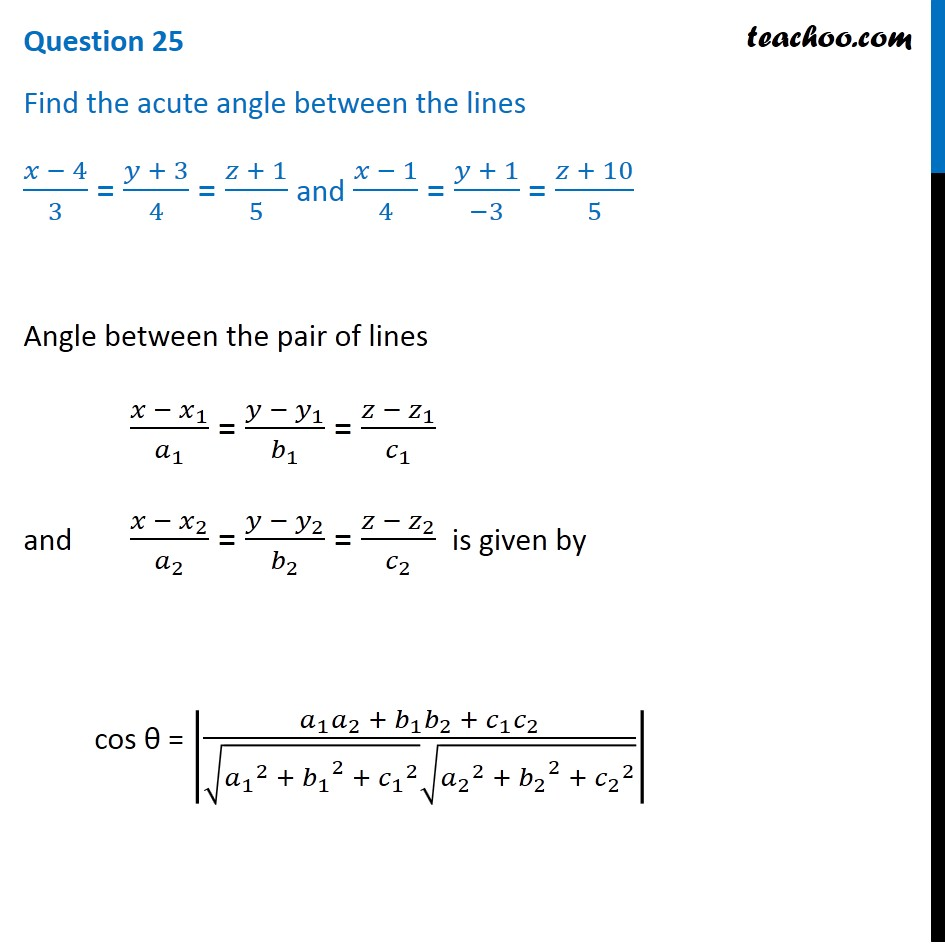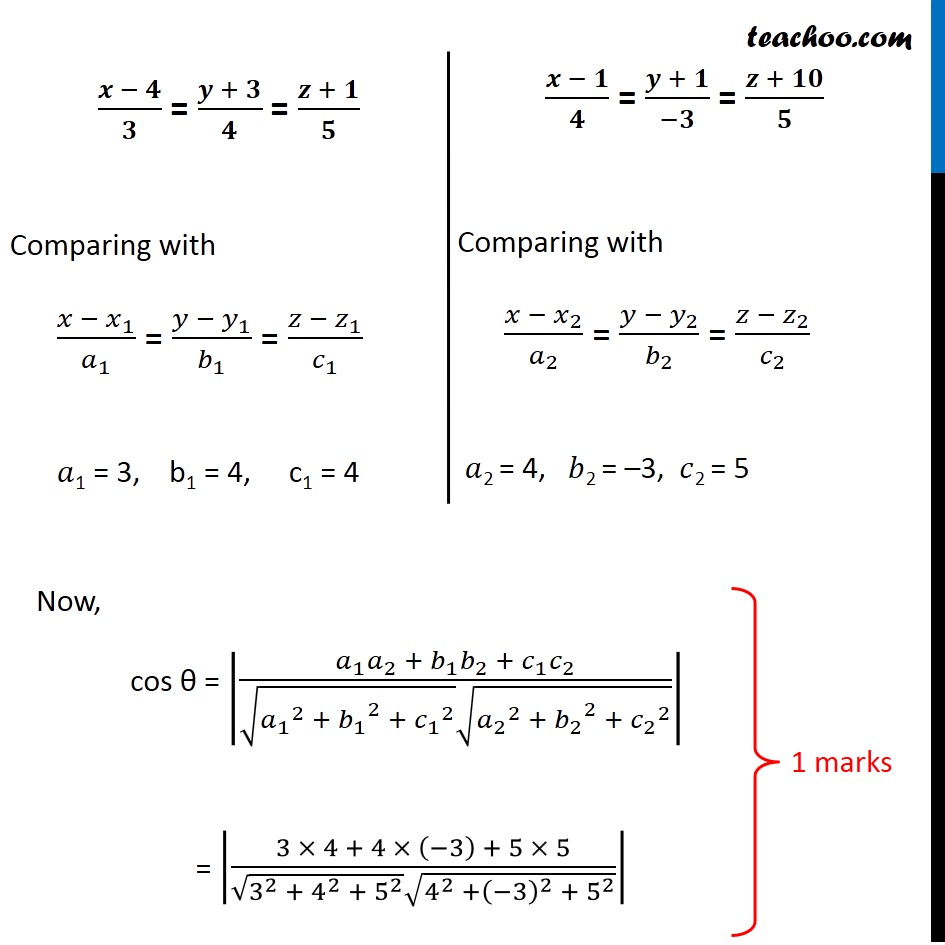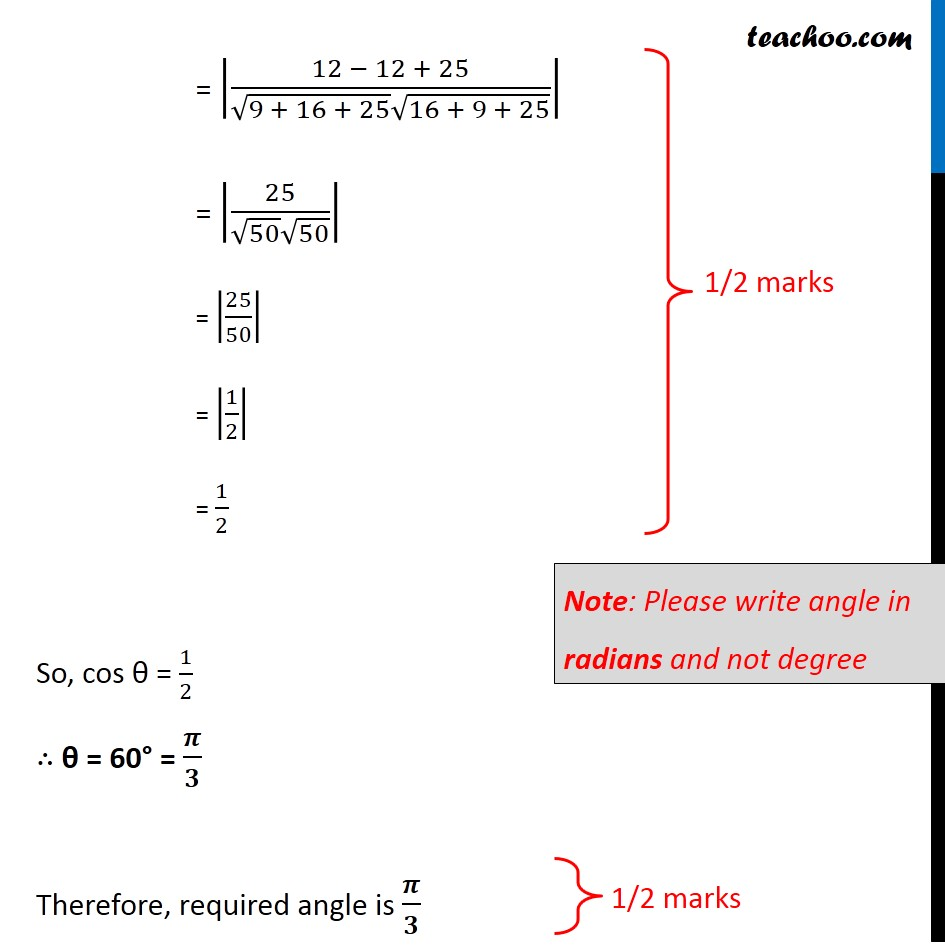## (x - 4)/3 = (y + 3)/4 = (z + 1)/5 and (x - 1)/4 = (y + 1)/(-3) = (z + 10)/51. Class 12
2. Solutions of Sample Papers and Past Year Papers - for Class 12 Boards
3. CBSE Class 12 Sample Paper for 2020 Boards

Transcript

Question 25 Find the acute angle between the lines (𝑥 − 4)/3 = (𝑦 + 3)/4 = (𝑧 + 1)/5 and (𝑥 − 1)/4 = (𝑦 + 1)/(−3) = (𝑧 + 10)/5 Angle between the pair of lines (𝑥 − 𝑥_1)/𝑎_1 = (𝑦 − 𝑦_1)/𝑏_1 = (𝑧 − 𝑧_1)/𝑐_1 and (𝑥 − 𝑥_2)/𝑎_2 = (𝑦 − 𝑦_2)/𝑏_2 = (𝑧 − 𝑧_2)/𝑐_2 is given by cos θ = |(𝑎_1 𝑎_2 + 𝑏_1 𝑏_2 + 𝑐_1 𝑐_2)/(√(〖𝑎_1〗^2 + 〖𝑏_1〗^2 + 〖𝑐_1〗^2 ) √(〖𝑎_2〗^2 + 〖𝑏_2〗^2 + 〖𝑐_2〗^2 ))| (𝒙 − 𝟒)/𝟑 = (𝒚 + 𝟑)/𝟒 = (𝒛 + 𝟏)/𝟓 Comparing with (𝑥 − 𝑥_1)/𝑎_1 = (𝑦 − 𝑦_1)/𝑏_1 = (𝑧 − 𝑧_1)/𝑐_1 𝑎1 = 3, b1 = 4, c1 = 4 (𝒙 − 𝟏)/𝟒 = (𝒚 + 𝟏)/(−𝟑) = (𝒛 + 𝟏𝟎)/𝟓 Comparing with (𝑥 − 𝑥_2)/𝑎_2 = (𝑦 − 𝑦_2)/𝑏_2 = (𝑧 − 𝑧_2)/𝑐_2 𝑎2 = 4, 𝑏2 = –3, 𝑐2 = 5 Now, cos θ = |(𝑎_1 𝑎_2 + 𝑏_1 𝑏_2 + 𝑐_1 𝑐_2)/(√(〖𝑎_1〗^2 + 〖𝑏_1〗^2 + 〖𝑐_1〗^2 ) √(〖𝑎_2〗^2 + 〖𝑏_2〗^2 + 〖𝑐_2〗^2 ))| = |(3 × 4 + 4 × (−3) + 5 × 5)/(√(3^2 + 4^2 + 5^2 ) √(4^2 +(−3)^2 + 5^2 ))| = |(12 − 12 + 25)/(√(9 + 16 + 25) √(16 + 9 + 25))| = |25/(√50 √50)| = |25/50| = |1/2| = 1/2 So, cos θ = 1/2 ∴ θ = 60° = 𝝅/𝟑 Therefore, required angle is 𝝅/𝟑 Note: Please write angle in radians and not degree

CBSE Class 12 Sample Paper for 2020 Boards

Class 12
Solutions of Sample Papers and Past Year Papers - for Class 12 Boards